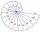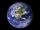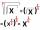Balls

Three metal balls with volumes V1=71 cm3 V2=78 cm3 and V3=64 cm3 melted into one ball. Determine it's surface area.

Result

S =  172.5 cm2

Solution:Leave us a comment of example and its solution (i.e. if it is still somewhat unclear...):Be the first to comment!Next similar examples:

1. Plasticine ballPlasticine balls have radius r1=85 cm, r2=60 mm, r3=59 cm, r4=86 cm, r5=20 cm, r6=76 mm, r7=81 mm, r8=25 mm, r9=19 mm, r10=14 cm. For these balls.
2. Theorem proveWe want to prove the sentence: If the natural number n is divisible by six, then n is divisible by three. From what assumption we started?
3. GasholderThe gasholder has spherical shape with a diameter 20 m. How many m3 can hold in?
4. Hemispherical hollowThe vessel hemispherical hollow is filled with water to a height of 10 cm =. How many liters of water are inside if the inside diameter of the hollow is d = 28cm?
5. Fit ballWhat is the size of the surface of Gymball (FIT - ball) with a diameter of 65 cm?
6. Sphere fallHow many percent fall volume of sphere if diameter fall 10×?
7. Sphere growthHow many times grow volume of sphere if diameter rises 10×?
8. Sphere A2VSurface of the sphere is 241 mm2. What is its volume?
9. Cylinder - A&VThe cylinder has a volume 1287. The base has a radius 10. What is the area of surface of the cylinder?
10. Volume of ballFind the volume of a volleyball that has a radius of 4 1/2 decimeters. Use 22/7 for π
11. PowersExpress the expression ? as the n-th power of the base 10.
12. CalculationHow much is sum of square root of six and the square root of 225?
13. One halfOne half of ? is: ?
14. PowerNumber ?. Find the value of x.
15. RootUse law of square roots roots: ?
16. Earth's surfaceThe greater part of the earth's surface (r = 6371 km) is covered by oceans; their area is approximately 71% of the Earth's surface. What is the approximate area of the land?
17. Square rootsWhat is equal to the product of the square roots of 295936?